• Call Now

1800-102-2727•

# Centripetal and centrifugal force - Examples, Differences, Practice problems, FAQs

Fathima is doing her laundry on a weekend. She loads her clothes in the washing machine and sets the timer. The machine starts to spin, removing all the dirt. She starts to wonder what is the mechanism behind this – how exactly was the dirt getting removed? At the same time, she notices her baby sister Sana playing with a stone toy. Sana is spinning the stone with a thread attached to it. In order to understand the physics behind the stone swirling in a circular path and the dirt getting removed from clothes, let us try to understand the concept of circular motion. Just like a typical 1D or 2D motion, circular motion could be uniform or non-uniform. If the speed remains constant throughout , it is termed a uniform motion. On the other hand, a non-uniform circular motion is one in which the speed does not remain the same throughout. For instance, baby Sana’s whirling toy is an example of non-uniform motion. In order for the particle in question to stay in circular motion, a centripetal force is needed to hold it in a circle. In other words, without the centripetal force, it would fly away from the circular path and become a projectile. Now according to Newton’s third law, an equal and opposite force must exist—this is nothing but the centrifugal force. The latter is what is responsible for removing dirt from clothes. In this article, we will explore centripetal and centrifugal force in detail.

• Definition of centripetal force
• Definition of centrifugal force
• Practice problems
• FAQs

## Definition of centripetal force

The force directed towards the center when an object is moving in a circular path of radius r with speed v, is called centripetal force (Fc). The force is directed radially towards the center of the circle. Let ac be the centripetal acceleration and at be the tangential acceleration.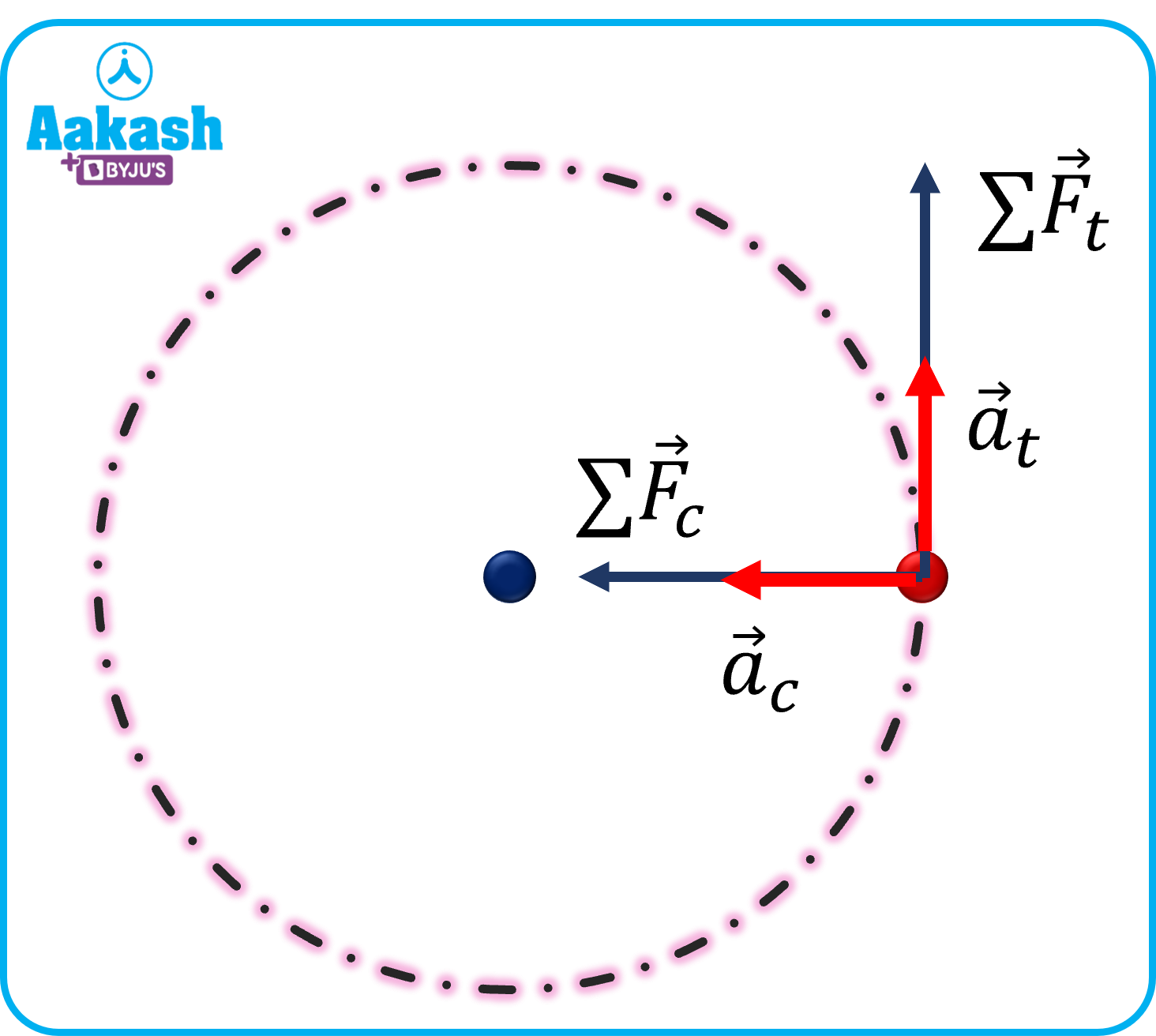By Newton’s second law, we have

$m{a}_{c}=\frac{m{v}^{2}}{r}$

The sum of all the forces ⅀mac=⅀Fc.

Examples of centripetal force:

(i) Electromagnetic force between the protons in the nucleus and the electrons revolving in orbits around the nucleus.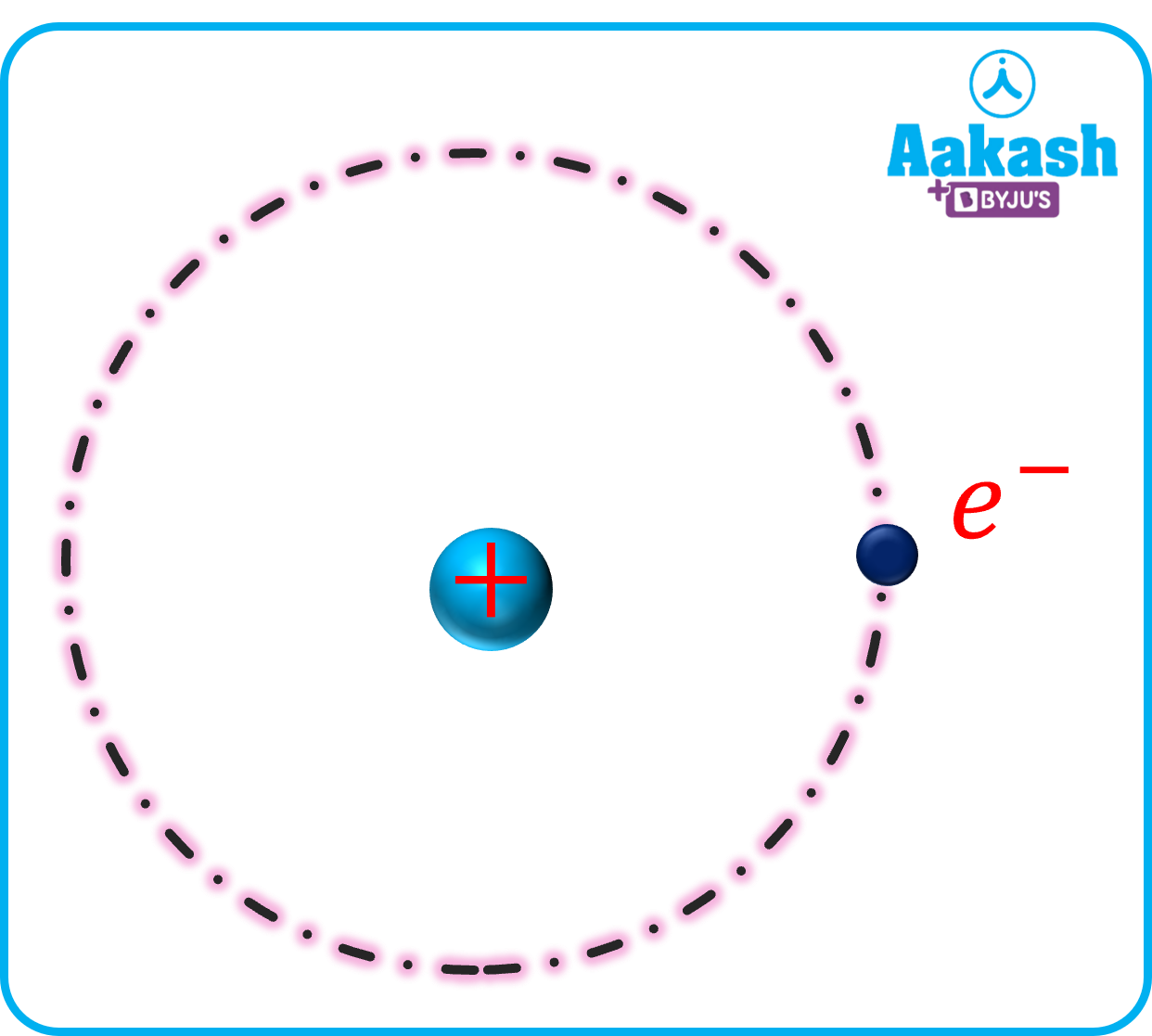(ii) Movement of a car on a circular track - The frictional force between the road and the tyres provides the necessary centripetal force.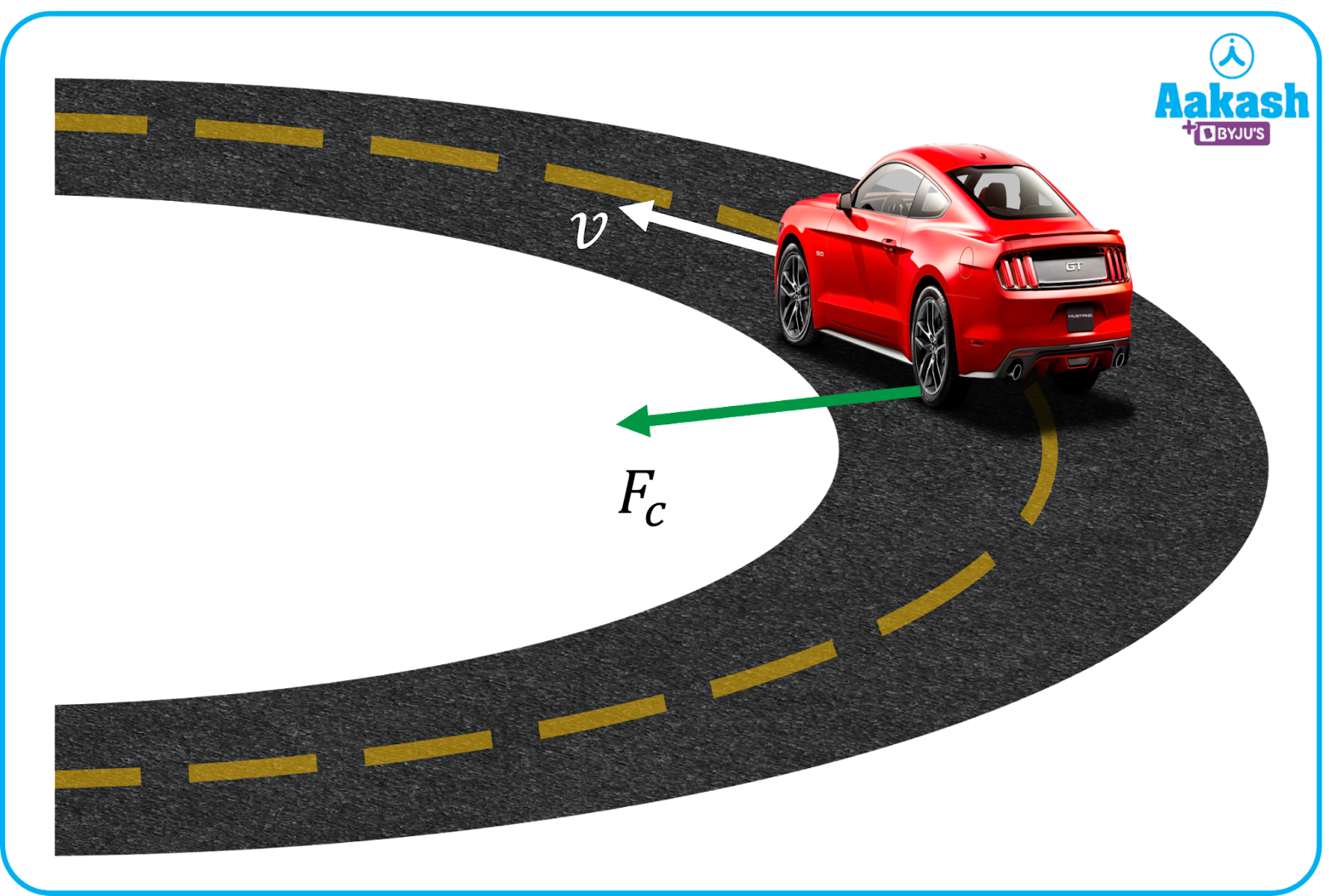Note:

In case of a circular motion, the velocity and centripetal force are perpendicular to each other.

Hence the power delivered by the force=Fcv cos 900=0 work done by centripetal force= zero.

## Definition of centrifugal force

When a body moves in a circular path, in the frame where the body is, the force that acts radially outward is called centrifugal force. It is also termed a pseudo or fictitious force. It comes into play only in non-inertial frames–ones in which Newton’s laws are not obeyed. Consider the following example where a cylinder of mass m, radius r is rotated with an angular speed . It is an example of a non-inertial frame– since the observers are rotating with some acceleration.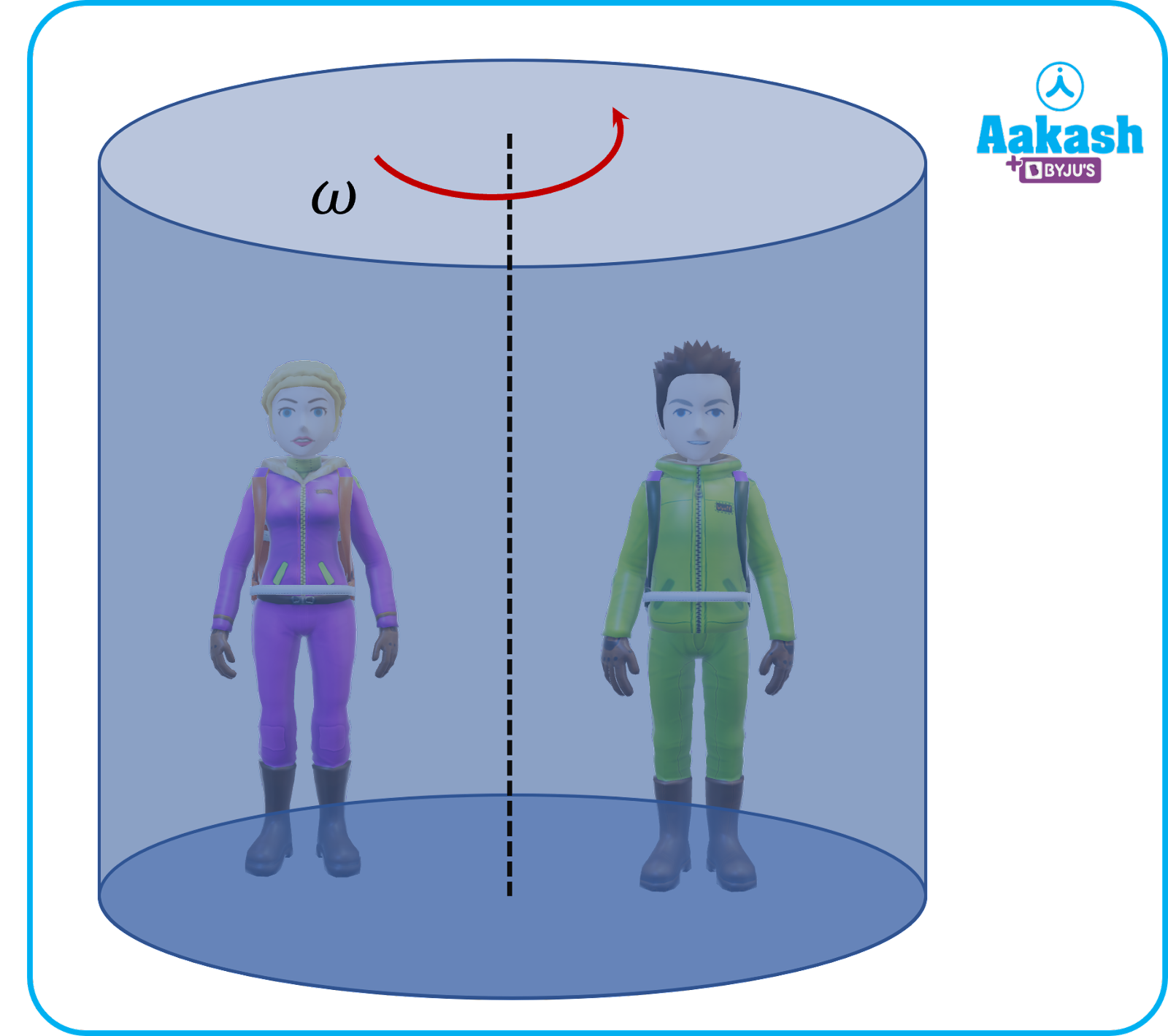The person is stuck on the wall of a rotating frame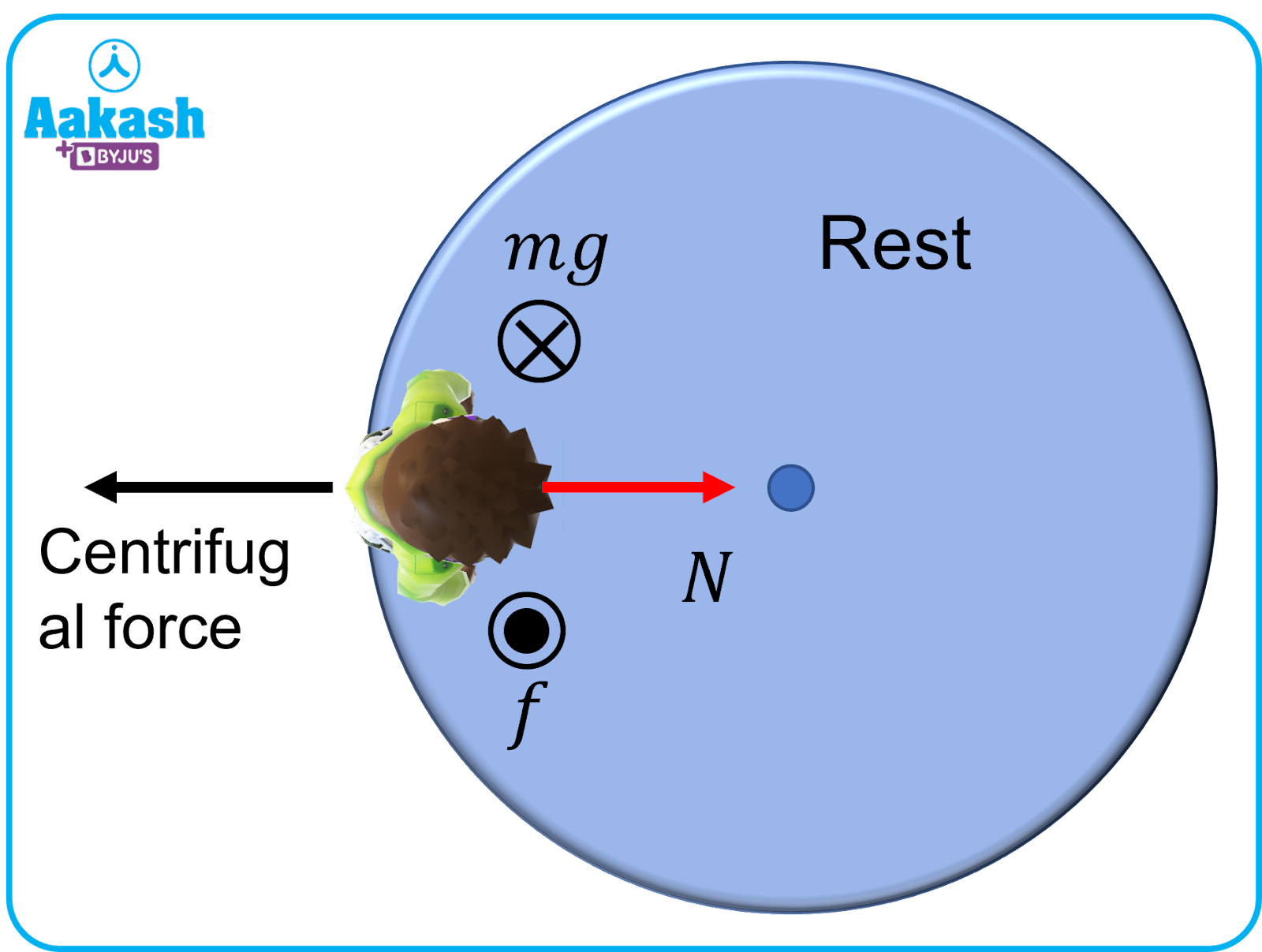Balancing forces in the horizontal and vertical directions, we get

Normal reaction, N=m2r; m2r indicates the centrifugal force.

Frictional force ,f=mg

Let fmax indicate the limiting value(maximum value) of friction.

Then for the cylinder to be in equilibrium,

is the coefficient of friction between the surface and the person.

$mg\le \mu \left(m{\omega }^{2}r\right)⇒\omega \ge \sqrt{\frac{g}{\mu r}}$

## Practice problems

Q.A stone of mass 2 kg is tied to a string of length 0.5 m. If the maximum breaking tension in the string is 900 N. What is the maximum angular velocity of the stone so that it stays in motion?

A. a

Given,

Mass of the string, m=2 kg.

Max. tension in the string, Tmax=900 N,=?

The tension provides the necessary centripetal force.

${\omega }^{2}=900;$

Q The radius of curvature of the path followed by two particles having same mass is in the ratio 1:2. For the particles to have the same centripetal force, what should be the ratio of their velocities?

(a)1:4 (b)4:1 (c)2:1 (d)1:2

A. d

Let m be the mass of the particle. Let r1 and r2 be the radii of the two particles.

Then,

$\frac{{r}_{1}}{{r}_{2}}=\frac{1}{2}$

Let v1 and v2 be the velocities of the two particles.

m(v1)2r1=m(v2)2r2v12v22=r1r2

v12v22=12;v1v2=12

Q.Two particles are revolving in a circular path of radius r1 and r2 respectively, with the same angular speed. What is the ratio of the centripetal forces on them if their masses are the same?

(a)r1r2 (b)r1r2 (c) (r1r2)2 (d)(r2r1)2

A. a

Ratio of the centripetal forces,

F1F2=mr12mr22=r1r2

Q.A proton having mass 1.67 10-27 kg goes in a circular orbit of radius 0.10 m under a centripetal force of 410-13 N. What is the frequency of revolution of proton?

A.

Mass of the proton, m=1.67 10-27 kg

Centripetal force, Fc=410-13 N

Radius of the orbit, r=0.10 m.

$F_c=\left\{\left\{mr\omega^2;\right\}_\right\}^$

Frequency,

## FAQs

Q. Does centrifugal force increase with speed?
A.
Since centrifugal force ${F}_{c}=m{\omega }^{2}r;i$ increasing the angular velocity increases the centrifugal force.

Q. How is centripetal force used in everyday life?
A.
Spinning of a merry go round or twirling of a lasso are everyday applications of centripetal force.

Q. Would gravity change if the earth stopped spinning?
A.
If the earth stopped spinning, you wouldn’t be suddenly thrown into space; the gravity will still hold you on the ground.

Q. Does centrifugal force act inside or out?
A.
Centrifugal force acts outwards.Talk to our expert
Resend OTP Timer =
By submitting up, I agree to receive all the Whatsapp communication on my registered number and Aakash terms and conditions and privacy policy